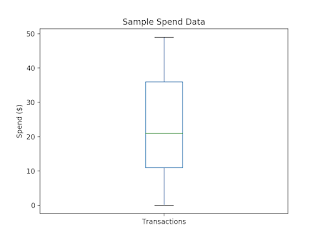# Analysis Toolkit: Robust One-Dimensional Statistics and Spend Data

## NOTE: For best viewing experience, use Chrome. There is one thing that is ubiquitous among procurement: data. No matter what category you are shopping for, purchases you are evaluating, or event you are analyzing, you will have to deal with it. However, more often than not, data is dirty. You will come across typos, automation mistakes, and computational mistakes on a regular basis. It is for this reason that one should develop a fundamental understanding around data quality and the techniques that should be used to inspect it. In what follows we will construct a data set to analyze. Once we have done this we will discuss quick inspection techniques and the difference between some of the well known metrics that one should use, like the mean and standard deviation, and some of the more robust measures that are often neglected like median and median absolute deviation. All code that follows was constructed in Python 2.7.

We begin be constructing a random sample of 100 integers with a maximum value of 50. These values will be used to represent spend. We may think of these as individual transactions for a company that does not exceed expenditures of more than 50 per purchase. We then add one value to our dataset intended to represent a maverick spend.

## GENERATE DATA

```    # generate sample data
n = 100
max_spend = 50
spend = data(size=n, max=max_spend)

spend.append(max_spend * 20)```

In order to gain a better understanding of our data we plot it. From both the dotplot and box-and-whiskers plot we can immediately see that there is a significant outlier.

## INSPECT DATA

```    # visualize data
spend.dotplot()
spend.whiskers()

# dimensionality reduction
spend.describe()
spend.describe_robust()```Alternatively, we can reduce the dimensionality of our dataset by running the inbuilt describe function and our self-built robust describe function. The combination of these two functions generate the following results:

count      101.00000
mean       32.178218
std        98.367210
median      21.00000
min          0.00000
25%         11.00000
50%         21.00000
75%         36.00000
max       1000.00000

It is important to note that the mean is quite a bit higher than the median. Furthermore, the standard deviation is significantly higher than the median absolute deviation.

## REMOVE OUTLIERS & REINSPECT DATA

We now perform outlier removal. There are a lot of methodologies for removing outliers, including but not limited to trimming and censoring. For demonstration purposes, we will implement both Winsorization and clipping. The former is the act of replacing the outlier(s) with data points estimated from the non-outlying data. Alternatively, clipping is the act of simply removing the outliers.

```    # remove outliers
spend.winsorize(0.01)
# visualize data
spend.dotplot()
spend.whiskers()

# dimensionality reduction
spend.describe()
spend.describe_robust()```

Note that when we plot the data after Winsorization, we no longer have the unusable graphics from before.Here we see that the Winsorized mean and Winsorized median are approximately the same and the Winsorized standard deviation and Winsorized median absolute deviation are approximately the same.

count    101.000000
mean      22.762376
std       14.919885
median     21.000000
min        0.000000
25%       11.000000
50%       21.000000
75%       36.000000
max       49.000000

Furthermore, these values are all approximately equal to the values of the median and median absolute deviation that we generated prior to removing our maverick spend. The takeaway: median and median absolute deviation are more robust statistics than mean and standard deviation because they are more resistant to outliers.

Alternatively, let's try clipping the outlier. Once we complete this exercise we again see that the clipped mean and clipped median are approximately the same and the clipped standard deviation and clipped median absolute deviation are approximately the same.

count     100.00
mean      22.500000
std       14.759007
median     20.50
min         0.00
25%        11.00
50%        20.50
75%        36.00
max        49.00

We also observe that the counts and point statistics are actually different for the Winsorized data and the clipped data. Hence, the choice of outlier removal could potentially play a big part in the actionable outcomes of our analysis. It is for this reason that not only should every procurement analyst be familiar with these methodologies, but they should use them intelligently when dealing with quantitative data. A worthwhile exercise for anyone uncomfortable with the above content would be to change the underlying distribution of the randomly generated numbers and see what the outcomes of the subsequent analysis would be. Specifically, how a different distribution could possibly affect the disparity of the non-robust metrics.

## COMPLETE CODE

```import numpy as np
import pandas as pd
import matplotlib.pyplot as plt
from scipy.stats.mstats import winsorize

class data(pd.Series):
def __init__(self, size, min=0, max=2, seed=0):
np.random.seed(seed)
pd.Series.__init__(self, np.random.randint(min, max, size))

def append(self, x):
pd.Series.__init__(self, np.append(self._values, x))

def describe_robust(self):
_ = [self.count(), self.median(), self.mad(), self.min(),
self.quantile(0.25), self.quantile(0.50), self.quantile(0.75),
self.max()]
description = pd.Series(_, index=index)
return(description)

def capture_maverick(self, x):
return(self[self.zscore().abs()>x])

def winsorize(self, x):
return(pd.Series.__init__(self, winsorize(self, limits=x)))

def normalize(self):
return(self - self.mean()) / (self.max() - self.min())

def zscore(self):
return((self - self.mean()) / self.std())

def dotplot(self):
ax = self.plot(marker='.', title='Sample Spend Data',
label='Transaction ID')
ax.set_ylabel('Spend (\$)')
return(ax)

def whiskers(self):
ax = self.plot(kind='box', title='Sample Spend Data',
label='Transactions')
ax.set_ylabel('Spend (\$)')
return(ax)

if __name__ == '__main__':

# generate sample data
n = 100
max_spend = 50
spend = data(size=n, max=max_spend)

spend.append(max_spend * 20)

# visualize data
spend.dotplot()
spend.whiskers()

# dimensionality reduction
spend.describe()
spend.describe_robust()

# identify outliers
spend.zscore()
spend.capture_maverick(2)

# remove outliers
spend.winsorize(0.01)

# visualize data
spend.dotplot()
spend.whiskers()

# dimensionality reduction
spend.describe()
spend.describe_robust()```
###### Share To: